# Capacitor Mathematics

Below is an index of our mathematical discussion of capacitors:

Capacitive reactance

Capacitance of a transmission line (separate page)

Quality factor

Parallel-plate capacitance

Sheet capacitance

Capacitor resonances

Calculating charge storage capacitance (separate page, new for March 2007!

Capacitor ESR effects (separate page, new for February 2009!)

### Capacitive reactance

Use our reactance calculator if you are interested in this topic!

Capacitive reactance is the "imaginary" impedance of a capacitor expressed in ohms. Note the negative sign, which implies that on a Smith Chart, adding series capacitance tends to rotate your reflection coefficient counterclockwise. It's a function of frequency.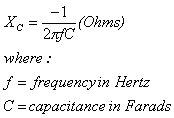Hey, Bill Gates, before we go any further with this page, we want to tell you that Microsoft Equation Editor 3.0 completely sucks! Now let's fix that formula for the more practical units of pico-Farads and GHz: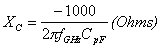### Quality factor

The quality factor is a measure of how lossless a capacitor is: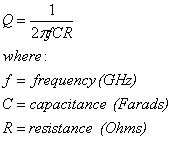Note that as you increase frequency, quality factor always gets worse (decreases). Let's put the equation into GHz and pico-Farads:Thus a 1 pF cap with one-tenth ohm resistance would have a Q of 159 at 10 GHz. Dissipation factor is merely the reciprocal of the quality factor: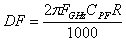The dissipation of the same 1 pF cap at 10 GHz would be 0.6%.

### Parallel-plate capacitance

The well-known formula for parallel-plate capacitors of "infinite size" is given below. Most parallel plate capacitors behave close to ideally because there area dimensions (length and width) are much bigger than their plate separation. No consideration of the fringing fields is usually necessary unless you are working with extremely small capacitors (perhaps less than 1 picofarad).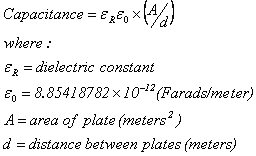This is a case where microwave engineering prefers the metric system, because the permittivity of free space is always expressed in Farads per meter, not Farads per inch. Because practical microwave circuits are much smaller than meters and Farads, we will rewrite the capacitance formula using millimeters and pico-Farads: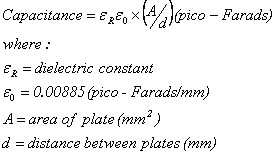### Sheet capacitance

Sheet capacitance is a useful simplification of the capacitor formula, if you are always using a given dielectric and thickness to construct capacitors (such as on a MMIC):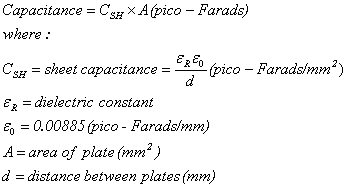Thus, if you are working with a MMIC foundry that offers 2000 Angstroms (0.2 micron) silicon nitride (er=7.5) for thin-film capacitors, the sheet capacitance is:

7.5 x 0.00885/0.0002=332 pF/mm2

If you construct a capacitor of 100 x 100 microns (0.1 x 0.1 mm), it will have a value of 3.3 pF.

### Capacitor resonances

The first resonance of a capacitor is the series resonant frequency. Referring to the model below, this is the frequency where the capacitive reactance and inductive reactance due to LS cancel.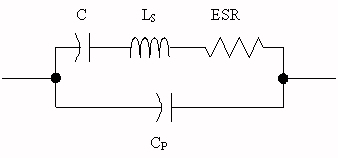Series resonant frequency (SRF)
The frequency at which the series inductance of a capacitor is equal but opposite to its capacitance. Click here for an explanation of series resonance on our filter page. This is where the capacitor behaves as low-value resistor (equal to the ESR value).

Most capacitor vendors tell you the series resonant frequency, not the inductance LS. To determine LS in nano-Henries, use the following equation (fixed on February 3, 2006!):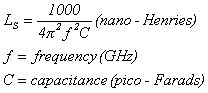Parallel resonant frequency (PRF)
This typically occurs at twice the SRF frequency. Click here for an explanation of parallel resonance on our filter page. Usually you have no business operating a capacitor at or near the PRF, because it acts like an open circuit in this case!

That's all for now!

Author : Unknown Editor